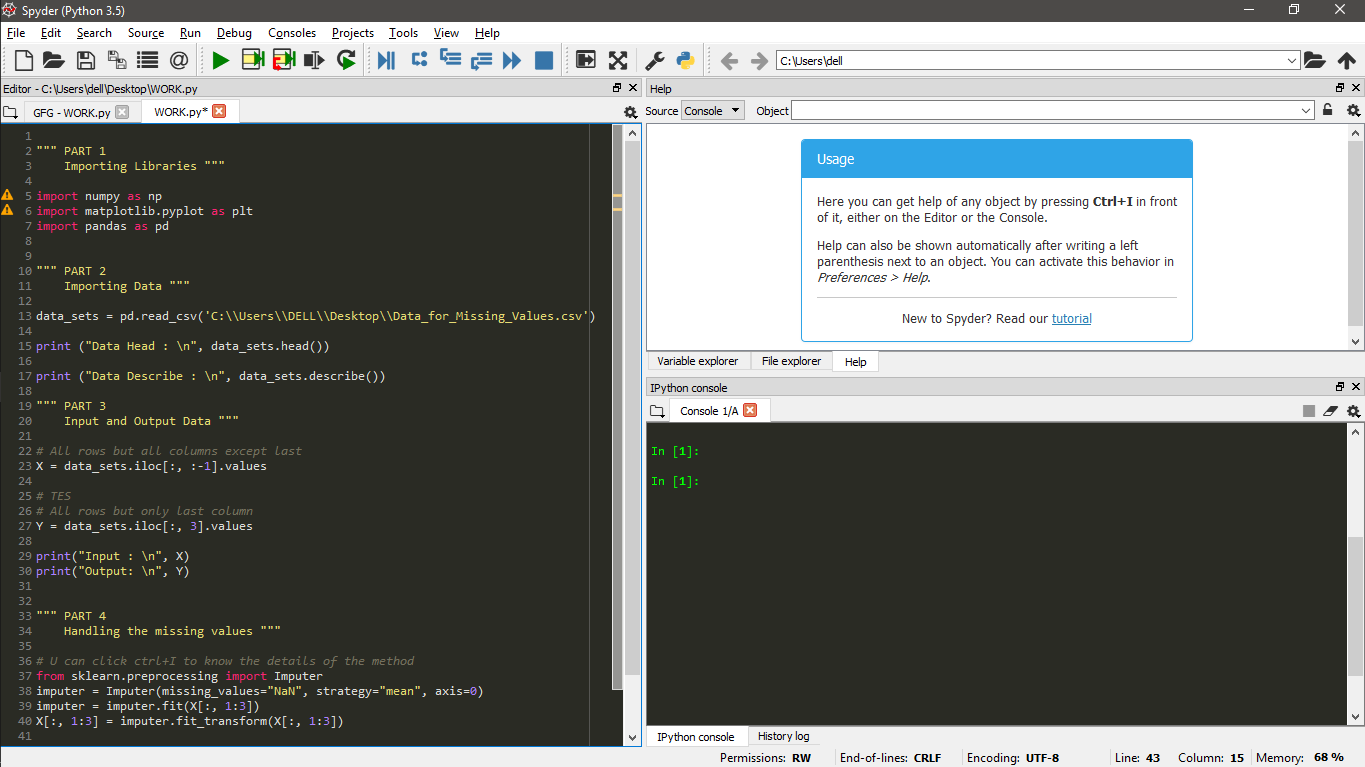Related Articles
ML | Handling Missing Values
• Last Updated : 16 Oct, 2019

To work with ML code, libraries play a very important role in Python which we will study in details but let see a very brief description of the most important ones :

• NumPy (Numerical Python) : It is one of the greatest Scientific and Mathematical computing library for Python. Platforms like Keras, Tensorflow have embedded Numpy operations on Tensors. The feature we are concerned with its power and easy to handle and perform operation on Array.
• Pandas : This package is very useful when it comes to handle data. This makes it very easier to manipulate, aggregate and visualize data.
• MatplotLib : This library facilitates the task of powerful and very simple visualizations.

There are many more libraries but they have no use right now. So, let’s begin.

Anaconda :
I would suggest you guys to install Anaconda on your systems. Launch Spyder our Jupyter on your system. Reason behind suggesting is – Anaconda has all the basic Python Libraries pre installed in it.Below is the Python code :

 `# Python code explaining How to``# Handle Missing Value in Dataset`` ` `""" PART 1``    ``Importing Libraries """`` ` `import` `numpy as np``import` `matplotlib.pyplot as plt``import` `pandas as pd`` ` ` ` `""" PART 2``    ``Importing Data """`` ` `data_sets ``=` `pd.read_csv(``'C:\\Users\\Admin\\Desktop\\Data_for_Missing_Values.csv'``)`` ` `print` `(``"Data Head : \n"``, data_sets.head())`` ` `print` `(``"\n\nData Describe : \n"``, data_sets.describe())`` ` `""" PART 3``    ``Input and Output Data """`` ` `# All rows but all columns except last``X ``=` `data_sets.iloc[:, :``-``1``].values`` ` `# TES``# All rows but only last column ``Y ``=` `data_sets.iloc[:, ``3``].values``                 ` `print``(``"\n\nInput : \n"``, X)``print``(``"\n\nOutput: \n"``, Y)`` ` ` ` `""" PART 4``    ``Handling the missing values """`` ` `# We will use sklearn library >> preprocessing package``# Imputer class of that package``from` `sklearn.preprocessing ``import` `Imputer`` ` `# Using Imputer function to replace NaN``# values with mean of that parameter value``imputer ``=` `Imputer(missing_values ``=` `"NaN"``,``                  ``strategy ``=` `"mean"``, axis ``=` `0``)``                   ` `# Fitting the data, function learns the stats``imputer ``=` `imputer.fit(X[:, ``1``:``3``])`` ` `# fit_transform() will execute those``# stats on the input ie. X[:, 1:3]``X[:, ``1``:``3``] ``=` `imputer.fit_transform(X[:, ``1``:``3``])`` ` `# filling the missing value with mean``print``(``"\n\nNew Input with Mean Value for NaN : \n"``, X)`

Output :

```Data Head :
Country   Age   Salary Purchased
0   France  44.0  72000.0        No
1    Spain  27.0  48000.0       Yes
2  Germany  30.0  54000.0        No
3    Spain  38.0  61000.0        No
4  Germany  40.0      NaN       Yes

Data Describe :
Age        Salary
count   9.000000      9.000000
mean   38.777778  63777.777778
std     7.693793  12265.579662
min    27.000000  48000.000000
25%    35.000000  54000.000000
50%    38.000000  61000.000000
75%    44.000000  72000.000000
max    50.000000  83000.000000

Input :
[['France' 44.0 72000.0]
['Spain' 27.0 48000.0]
['Germany' 30.0 54000.0]
['Spain' 38.0 61000.0]
['Germany' 40.0 nan]
['France' 35.0 58000.0]
['Spain' nan 52000.0]
['France' 48.0 79000.0]
['Germany' 50.0 83000.0]
['France' 37.0 67000.0]]

Output:
['No' 'Yes' 'No' 'No' 'Yes' 'Yes' 'No' 'Yes' 'No' 'Yes']

New Input with Mean Value for NaN :
[['France' 44.0 72000.0]
['Spain' 27.0 48000.0]
['Germany' 30.0 54000.0]
['Spain' 38.0 61000.0]
['Germany' 40.0 63777.77777777778]
['France' 35.0 58000.0]
['Spain' 38.77777777777778 52000.0]
['France' 48.0 79000.0]
['Germany' 50.0 83000.0]
['France' 37.0 67000.0]]
```

CODE EXPLANATION :

• Part 1 – Importing Libraries : In the above code, imported numpy, pandas and matplotlib but we have used pandas only.
• PART 2 – Importing Data :
• Import `Data_for_Missing_Values.csv` by giving the path to pandas read_csv function. Now “data_sets” is a DataFrame(Two-dimensional tabular data structure with labeled rows and columns).
• Then print first 5 data-entries of the dataframe using head() function. Number of entries can be changed for e.g. for first 3 values we can use dataframe.head(3). Similarly, last values can also be gotten using tail() function.
• Then used describe() function. It gives statistical summary of data which includes min, max, percentile (.25, .5, .75), mean and standard deviation for each parameter values.
• PART 3 – Input and Output Data : We split our dataframe to input and output.
• PART 4 – Handling the missing values : Using Imputer() function from sklearn.preprocessing package.

IMPUTER :
`Imputer(missing_values=’NaN’, strategy=’mean’, axis=0, verbose=0, copy=True)` is a function from Imputer class of sklearn.preprocessing package. It’s role is to transformer parameter value from missing values(NaN) to set strategic value.

```Syntax : sklearn.preprocessing.Imputer()

Parameters :

-> missing_values  : integer or “NaN”
-> strategy        : What to impute - mean, median or most_frequent along axis
-> axis(default=0) : 0 means along column and 1 means along row
```My Personal Notes arrow_drop_up